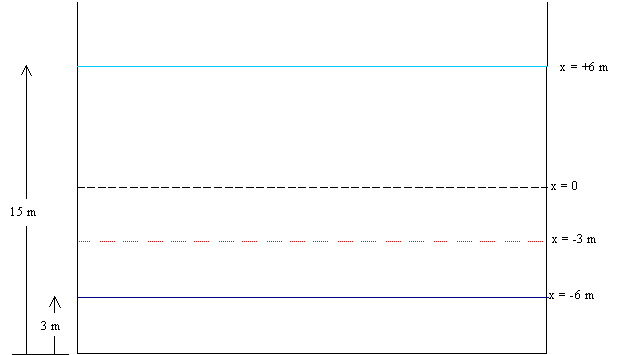Problem 1.

 The water level in a harbor can be assumed to rise and fall with simple harmonic motion. On a certain day, low tide will occur at 12 noon and the depth of the water will be 3M. The subsequent high tide will occur at 6:20pm and the water will then be 15M deep. A ship which needs 6M depth of water wishes to use the harbor. Find to the nearest minute the earliest time it can enter the harbor on this day and the time by which it must leave.

.The level of water from the see bottom are indicated in the left of the diagram and the equilibrium point of the simple harmonic motion (y=0) and the positions as measured from this point are shown on the right. The equation for the SHM with the initial condition y = -A (amplitude) at t =0 is given by:

y= -A cos{(2p/T) t}; the level y of the sea as a function of time t. I hope you understand that the y coordinate should have the origin at the equilibrium point to use this equation.

Now the red line is the critical sea level below which the ship shouldn’t enter the harbor (y = -3 m).

Recognize:

A = 6 m

T = 2*(06:20 pm - 12:00 noon) = 760 minutes

Now all we have to do is to substitute for y = -3 m in the equation and solve for t, as simple as that.

t =  T/2p cos-1(y/A)

Note that this equation will have multitude of answers, as there are many qs for

q = cos-1(y/A); also don’t forget that q should be in radian. But of course we can relate all the positive values of t to the real (physical) problem. The smallest positive value is when the sea level first reaches the y = -3 m, and the next smallest is when it reaches it for the second time etc.. If we designate those solutions as t1, t2, t3 and so on, it can be shown that t2 = T – t1 and t3 = T + t1, t4 = 2T – t1, t5 = 2T + t1 etc..

Below I use some JavaScript to solve this equation to get the required answers. Note that you can use the ‘calculator’ below to find the time t given the level y. Anyway please make sure you know how to use your own calculator to solve the SHM equation.

 Period, T (minutes) Amplitude, A (m) y (m) t1(minutes) t2(minutes)

If you fill the boxes with T = 760 minutes, A = 6 m, y = -3, you will get the solution t1 =  127 minutes and t2 = 633 minutes, which are the correct solutions. The ship has to get in before 02:07pm and leave before 10:33pm.